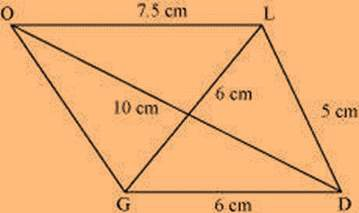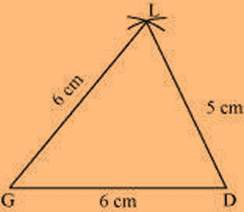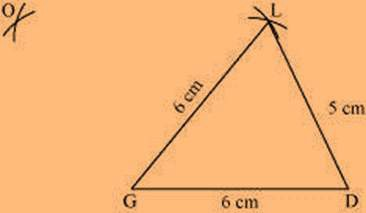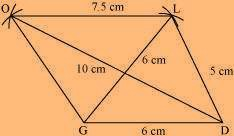# Construct the following quadrilateral: Quadrilateral GOLD. OL = 7.5 cm GL = 6 cm GD = 6 cm LD = 5 cm OD = 10 cm

Solution

The rough sketch of the quadrilateral GOLD can be drawn as follows.(1) ∆ GDL can be constructed by using the given measurements as per the steps given below(2) Vertex O is 10 cm away from vertex D and 7.5 cm away from vertex L.

Hence, while taking D and L as centres, draw arcs of 10 cm radius and 7.5 cm radius respectively.

These will intersect each other at point O.(3) Join O to G and L.